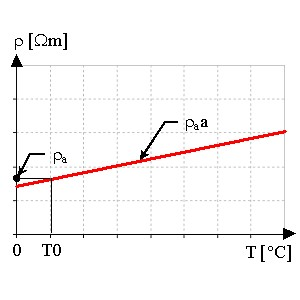# Resistivity, linear function of T

## Presentation

This model defines a resistivity ρ as a linear function of the temperature for an isotropic or anisotropic material.

## Mathematical model

Resistivity is a linear function of the temperature.

The corresponding mathematical formula is written:

ρ (T)= ρa(1+a(T - T0))

where:

• T0 is the reference temperature [degree]
• ρa is the resistivity of the material at T0 [Ω .m]
• a is a coefficient (positive or negative) [degree-1]

The shape of ρ(T) curve is presented in the figure below.## Anisotropic material

For an anisotropic material, this linear model consists of a group of three straight lines.